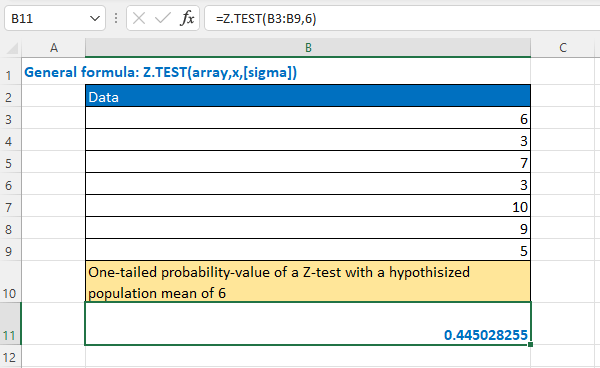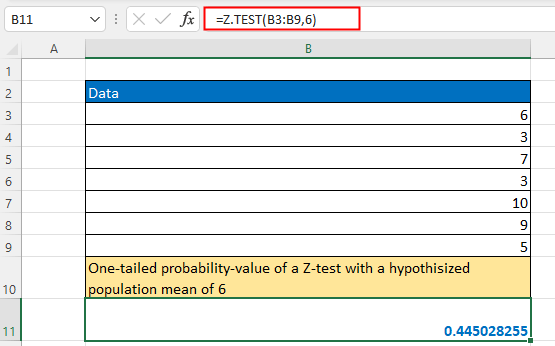## Excel Z.TEST Function### Description

The Excel Z.TEST function returns the one-tailed P-value of a z-test which is useful for various analyses. Based on the given hypothesized population mean, x, Z.TEST function returns the probability that the sample mean would be greater than the observed sample mean.

Formula syntax

Z.TEST(array,x,[sigma])

Arguments

 array: Required, the array or range of the data against which to test x. x: Required, the value used to test. sigma: Optional, represents to use the population’s standard deviation if this is known. If it is omitted, the sample standard deviation is used.

### Returns

Z.TEST function returns a numeric value.

### Remarks

1. Z.TEST represents the probability that the sample mean would be greater than the observed mean AVERAGE(array), when the underlying population mean is μ0. From the symmetry of the normal distribution, if AVERAGE(array) < x, Z.TEST returns a value greater than 0.5.

2. Z.TEST is calculated as follows

• when sigma is known:
Z.TEST( array,x,sigma ) = 1- Norm.S.Dist ((Average(array)- x) / (sigma/√n),TRUE)
• when sigma is omitted:
Z.TEST( array,x ) = 1- Norm.S.Dist ((Average(array)- x) / (STDEV(array)/√n),TRUE)
Where x is the sample mean AVERAGE(array), and n is COUNT(array).

### Errors

1. #VALUE! error – Occurs when the x or sigma is not a numeric value.

2. #NUM! error – Occurs when sigma = 0.

3. #N/A! error – Occurs when array is empty.

4. #DIV/0! error – Occurs when array contains only one value, or sigma is omitted and the standard deviation of the supplied array is 0.

### Version

Excel 2010 and later versions

### Usage and Examples

Basic usage

To get the one-tailed probability value of a z-test for the data in range B3:B9 with a hypothesized population mean of 6, please use the formula as this:

=Z.TEST(B3:B9,6)

Press Enter key to get the result.#### Other Functions:

• Excel POISSON Function
The Excel POISSON function returns the Poisson distribution which is used to predict the number of events occurring over a specific time.

• Excel WEIBULL Function
The Excel WEIBULL function returns the Weibull probability density function or Weibull cumulative distribution for a supplied set of parameters.

• Excel EXPON.DIST Function
EXPON.DIST function used in Excel 2010, returns the exponential distribution based on the given x value and the parameter of the distribution.

• Excel F.DIST Function
The Excel F.DIST function returns the F probability distribution which is usually used to measure the degree of diversity between two data sets.

### The Best Office Productivity Tools

#### Kutools for Excel - Helps You To Stand Out From Crowd

 Popular Features: Find/Highlight/Identify Duplicates  |  Delete Blank Rows  |  Combine Columns or Cells without Losing Data  |  Round without Formula ... Super VLookup: Multiple Criteria  |  Multiple Value   |  Across Multi-Sheets  |  Fuzzy Lookup... Adv. Drop-down List: Easy Drop Down List  |  Dependent Drop Down List  |  Multi-select Drop Down List... Column Manager: Add a Specific Number of Columns  |  Move Columns  |  Unhide Columns  |  Compare Columns to Select Same & Different Cells ... Featured Features: Grid Focus  |  Design View  |  Big Formula Bar  |  Workbook & Sheet Manager  |  Resource Library (Auto Text)  |  Date Picker  |  Combine Worksheets  |  Encrypt/Decrypt Cells  |  Send Emails by List  |  Super Filter  |  Special Filter (filter bold/italic/strikethrough...) ... Top 15 Toolset:  12 Text Tools (Add Text, Remove Characters ...)  |  50+ Chart Types (Gantt Chart ...)  |  40+ Practical Formulas (Calculate age based on birthday ...)  |  19 Insertion Tools (Insert QR Code, Insert Picture from Path ...)  |  12 Conversion Tools (Numbers to Words, Currency Conversion ...)  |  7 Merge & Split Tools (Advanced Combine Rows, Split Cells ...)  |  Many More...

Kutools for Excel Boasts Over 300 Features, Ensuring That What You Need is Just A Click Away...

Supports Office/Excel 2007-2021 and 365   |   Available in 44 Languages   |   Full-Featured 30-Day Free Trial.#### Office Tab - Enable Tabbed Reading and Editing in Microsoft Office (include Excel)

• One second to switch between dozens of open documents!
• Reduce hundreds of mouse clicks for you every day, say goodbye to mouse hand.
• Increases your productivity by 50% when viewing and editing multiple documents.
• Brings Efficient Tabs to Office (include Excel), Just Like Chrome, Firefox, And New Internet Explorer.